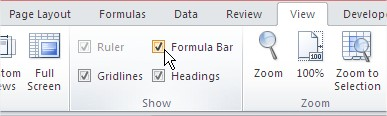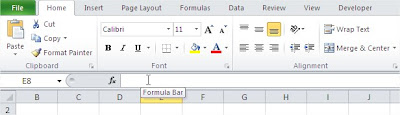### Missing Formula Bar In Excel

You can’t find a formula bar? In this lesson you can learn how to fix it.

Sometimes there is a simple problem in Excel. You can’t choose your formula, because the formula bar is missing.Where is it? Take a look how to get the formula bar back.

Go to the ribbon. View / Show and tick a Formula Bar.Formula Bar is back and you can to work on.Formula bar is not missing any more.

## Template

```Further reading: